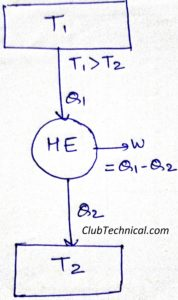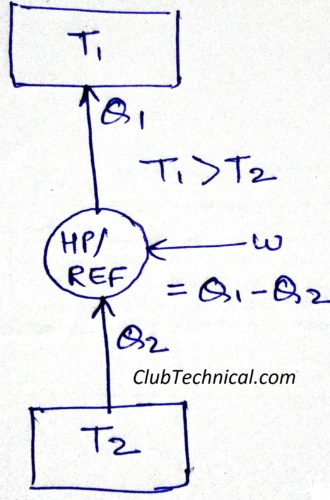# Efficiency and Coefficient of Performance (COP)

By | March 2, 2019

## Efficiency of a Heat Engine

A heat engine is a device which is used to convert heat into work. Efficiency of a heat engine is defined as the ratio of its work output and heat input.

Efficiency of a Heat Engine = Work output/ Heat inputHeat Engine

η = (Q1-Q2)/Q1 = 1 – (Q2/Q1)

For a reversible heat engine:

Q2/Q1 = T2/T1

For understanding above relation refer: Absolute thermodynamic scale of temperature

Hence for a reversible heat engine

ηREV = 1- (T2/T1)

## Coefficient of Performance (COP) of Heat pump and Refrigerator

Heat pump and refrigerator are two names of the same device.

When we use this device to remove heat then it is called a refrigerator and when we use this device to obtain heat then it is called a heat pump.

Coefficient of Performance (COP) is defined as the ratio of desired effect to work input.

COP = Desired effect/ Work input

In case of heat pump this desired effect is heating and in case of refrigerator this desired effect is cooling.Heat Pump or Refrigerator

1. COP of a Heat Pump

COPHP = Q1/Q1-Q2

COP for a reversible Heat Pump

COPHP REV = T1/T1-T2

1. COP of a Refrigerator

COPREF = Q2/Q1-Q2

COP for a reversible Refrigerator

COPREF REV = T2/T1-T2

By comparing COPs of Heat pump and Refrigerator we can easily observe that

COPHP = 1 + COPREF